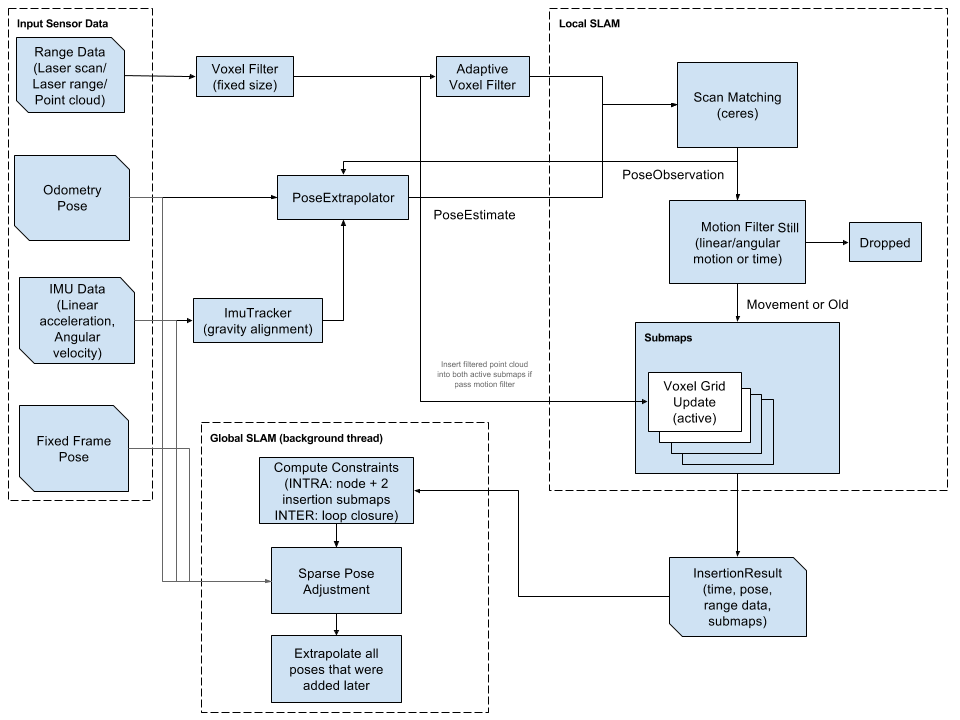## Wednesday, April 15, 2015

### A Kalman Filter for a Robot Car

This past week, I have spent quite a bit of time working on a simulator for a two-wheel differential drive robot.  The idea was to use simulated encoder and range finder data and an Extended Kalman filter to determine the location of a robot.  My implementation is written in Python and hosted here.

The system is modeled as having two inputs - The left and right wheel speeds.  The robot is externally given control inputs, but they are unknown to the Kalman filter.  The only inputs to the Kalman filter system are the encoder values and the range finder values and a set of known landmarks (this is to simulate mapping or some other landmark detection).  The encoder inputs are Vl and Vr.  From these values, we can surmise the average velocity V, and the rotational velocity w of the robot.
V = (Vl + Vr)/2
w = (Vr - Vl)/L, where L = length between wheels
We can then use the image below to determine the simple version of the equations of motion of the robot.An image of a differential drive robot.
From this system, we can surmise that the equations of motion, in discrete form, are:
x(k+1) = x(k) + V(k)*Cos(theta(k))*dt
y(k+1) = y(k) + V(k)*Sin(theta(k))*dt
theta(k+1) = theta(k)  + w(k)*dt
This means that we can assign the system states to be X, Y and Theta.  Because the system model above is ideal, we also need to add in a Gaussian noise value for each state. Now the system looks like this:
x(k+1) = x(k) + (V(k) + Nv) *Cos(theta(k))*dt
y(k+1) = y(k) + (V(k) + Nv) *Sin(theta(k))*dt
theta(k+1) = theta(k)  + (w(k) + Nw)*dt
The Kalman filter has two steps. The prediction step, and the update step.  The prediction step uses the system model, previous state values and control inputs (in this case, encoder values) to create a prediction (a priori) of the new states of the system.  It also creates an estimate covariance matrix, that is essentially a mathematical way to determine how much you trust the sensor values and the prediction.  The matrices needed for the calculation of the prediction step are the A, G, Q and P matrices.  A is the Jacobian, with respect to the system states, for the model shown above.  G is the Jacobian with respect to the noise values.  Q is a matrix built up by the covariances of the process noise.   The prediction step equations are:
X^(k+1) = f (X(k), U(k)) , where f (X(k), U(k)) is the original system model
P^(k+1) = A*P(k)*transpose(A) + G*Q*transpose(G)
The update step (a posteriori) is a bit more complicated.  The idea is to use a separate sensor (or multiple) to improve the quality of the prediction.  In this case, we will use a range finder to find landmarks and use those landmarks to determine where we should be and correct the position.  Of course, the range finder is not a perfect sensor and has Gaussian noise too.  This will be included in our calculations.  There are 4 parts of the update step.  The first is to calculate the difference in the measured values (from the range finder) and the predicted values from the previous step.  The h matrix, or measurement matrix, puts the states from the last step into the same space as the measurement values.  Then, we also need to calculate the Kalman gain.  This gain is a matrix that tells us how much we want to use the new measurement to update the prediction.  It is calculated using the sensor covariance matrix, the estimate covariance matrix and the Jacobian of the h matrix, H.  Using the residual and the Kalman Gain, we update the state prediction.  Finally, we updated the covariance matrix, using the Kalman Gain and H matrix.  The actual formulas are laid out below.
Y = Z - h(X(k))
K = P^(k+1)*H*(H*P^(k+1)*transpose(H) + R)^-1
X(k+1) = X^(k+1) + K*Y
P(k+1) = (I - K*H)*P^(k+1)
In order to make the landmark detection work, the x and y positions of the landmarks were augmented to the states matrix. X.  By adding those values, the Kalman Filter became an EKF and many other matrices had to be changed, as well.   The reason for doing this, is to keep track of the landmarks and allow them to make changes to the prediction, only if they are seen.  In my case, they are always seen, but in an real-world scenario, the range finder might not be able to see all of the landmarks or misses them.

Here are some results of my work.  A typical run can be seen below.  In this image, the actual position of the robot is in blue, the initial prediction is in green and the final prediction (after landmark detection with 20 landmarks) is in red.  The robot tracks the random path very well over 2 minutes, with a time step of 0.1 seconds.
The error between the actual and updated paths for this run can be seen below.  There was an average RMS error of 3.45 units over the course of the path.  What is neat, is that the Kalman Filter seems to correct itself when there is a lot of error. If odometry was the only sensor used, the error would grow much faster, and it would not correct itself.